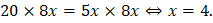# The following question consists of two statements I and II are given below. You have to decide whether the data provided in the statements are sufficient to answer the question. What is the average age of A and B? I. The ratio between one-fifth of A’s age and one-fourth of B’s age is 1:2. II. Product of their ages is 20 times B’s age. (a) The data in statement I alone was sufficient to the answer the question while II alone not sufficient to the answer the question. (b) The data in statement II alone are sufficient to answer the question while data in statement I alone are not sufficient to answer the question. (c) The data in statement I alone or in statement II alone are sufficient to answer the question. (d) The data in both statements I and II are  sufficient to answer the question.

## Question ID - 54485 :- The following question consists of two statements I and II are given below. You have to decide whether the data provided in the statements are sufficient to answer the question. What is the average age of A and B? I. The ratio between one-fifth of A’s age and one-fourth of B’s age is 1:2. II. Product of their ages is 20 times B’s age. (a) The data in statement I alone was sufficient to the answer the question while II alone not sufficient to the answer the question. (b) The data in statement II alone are sufficient to answer the question while data in statement I alone are not sufficient to answer the question. (c) The data in statement I alone or in statement II alone are sufficient to answer the question. (d) The data in both statements I and II are  sufficient to answer the question.

3537

I.II. 20B = AB.

Let A’s age beyrs. Then, B’s age =yrs.A = 20, B = 32.

Thus, I and II both together give the answer.

Next Question :
 The gravitational attraction between the two bodies increases when their masses are a) Reduced and distance is reduced b) Increased and distance is reduced c) Reduced and distance is increased d) Increased and distance is increased